Next: Normalization uncertainty Up: Matrice di covarianza di Previous: Matrice di covarianza di   Indice

### Offset uncertainty

Let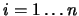be theresults of independent measurements and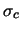the (diagonal) covariance matrix. Let us assume that they are all affected by the same calibration constant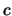, having a standard uncertainty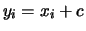. The corrected results are then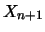. We can assume, for simplicity, that the most probable value ofis 0, i.e. the detector is well calibrated. One has to consider the calibration constant as the physical quantity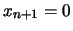, whose best estimate is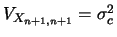. A term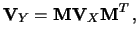must be added to the covariance matrix.

The covariance matrix of the corrected results is given by the transformation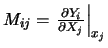(14.14)

where. The elements of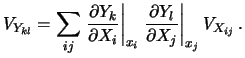are given by(14.15)

In this case we get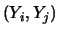(14.16) Cov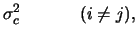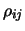(14.17)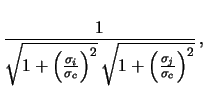(14.18)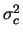(14.19)

reobtaining the results of Section. The total uncertainty on the single measurement is given by the combination in quadrature of the individual and the common standard uncertainties, and all the covariances are equal to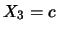. To verify, in a simple case, that the result is reasonable, let us consider only two independent quantities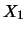and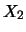, and a calibration constant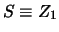, having an expected value equal to zero. From these we can calculate the correlated quantities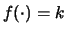and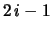and finally their sum (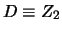) and difference (). The results are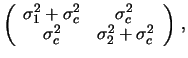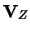(14.20)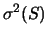(14.21)

It follows that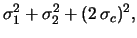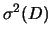(14.22)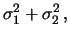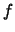(14.23)

as intuitively expected.Next: Normalization uncertainty Up: Matrice di covarianza di Previous: Matrice di covarianza di   Indice
Giulio D'Agostini 2001-04-02